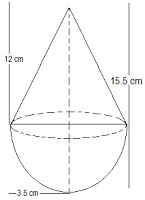# A toy is in the form of a cone of radius 3.5 cm mounted on a hemisphere of same radius. The total height of the toy is 15.5 cm. Find the total surface area of the toy.the radius of the cone and the hemisphere (r) = 3.5 cm or 7/2 cm

The total height of the toy is given as 15.5 cm.

So, the height of the cone (h) = 15.5-3.5 = 12 cm

l=√(h2+r2)

l=√(122+3.52)

l=√(144+12.25)

=√256.25

=12.5cm

Total surface area of toy=C.S. A of cone+ C.S.A of hemisphere

=πrl+2πr2

=πr(l+2r)

=22/7*3.5(12.5+2(3.5))

=11(12.5*7)

=11(19.5)

=214.5cm2